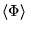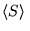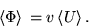# Energy flux in traveling waves

## Homework Statement

Two threads, both having linear density μ1 and μ2 are connected and tensed with tension T.
a) If traveling wave enters onto their junction, find ratios of amplitudes of reflected and input wave, transmitted and input wave, for μ21= 0, 1/4, 1, 4 and infinity
b)
Show that energy flow of input wave is equal to sum of reflected and transmitted wave.

## The Attempt at a Solution

As for part a, it is easy.
We have three waves;
Ψin = A cos (ωt - kz)
ψTr = T*A cos (wt -k2z)
ψRef = R*A cos (wt + kz)

R= (Z1-Z2)/(Z1+Z2), T= 1+ R
Z=(T0*μ)1/2
-> R=[(T01)1/2-(T02)1/2]/[(T01)1/2+(T02)1/2]=...=[(1-(μ21)1/2]/[(1+(μ21)1/2].
It is trivial to find those values now.

As for part b)
"The mean energy flux is, also written asand called I, the intensity. For a traveling wave,"
quoted from (http://scienceworld.wolfram.com/physics/EnergyFlux.html)
while i assume, U is potential energy.
So basicly, I need to find flux for those three waves, and prove statement.
How to find mean of U as for traveling waves?

haruspex
Homework Helper
Gold Member
2020 Award
Not sure of the exact formula, but for this question you only need to understand how the power in a wave depends on amplitude, frequency and density of medium. Consider a small element of length dx. Mass = ##\rho dx##. If its motion is given by ##y = A \sin(\omega t)##, what is its velocity at time t? What is its KE at time t? What is its average KE over time?
Once you have that, figure out the power per unit length in each of the three waves.

Velocity is derivative of y in respect to t -> v =dy/dt= wAcos(wt)
Kinetic energy is is 1/2 ρdx (dy/dt), so average is integral of that expression = .. = 1/4 ρ*A2*w2
(we loose cosine because average of cos squared is 1/2)

haruspex
Homework Helper
Gold Member
2020 Award
Velocity is derivative of y in respect to t -> v =dy/dt= wAcos(wt)
Kinetic energy is is 1/2 ρdx (dy/dt), so average is integral of that expression = .. = 1/4 ρ*A2*w2
(we loose cosine because average of cos squared is 1/2)
Good. Now, in the present case, w is the same for all three waves, so we can leave that out, and the 1/4, and get that it is k ρA2 for some constant k.
Substitute in the values of density and amplitude for each of the three waves. Do the transmitted and reflected power add up to the power of the incoming wave?

I agree. But they dont add up :/

haruspex